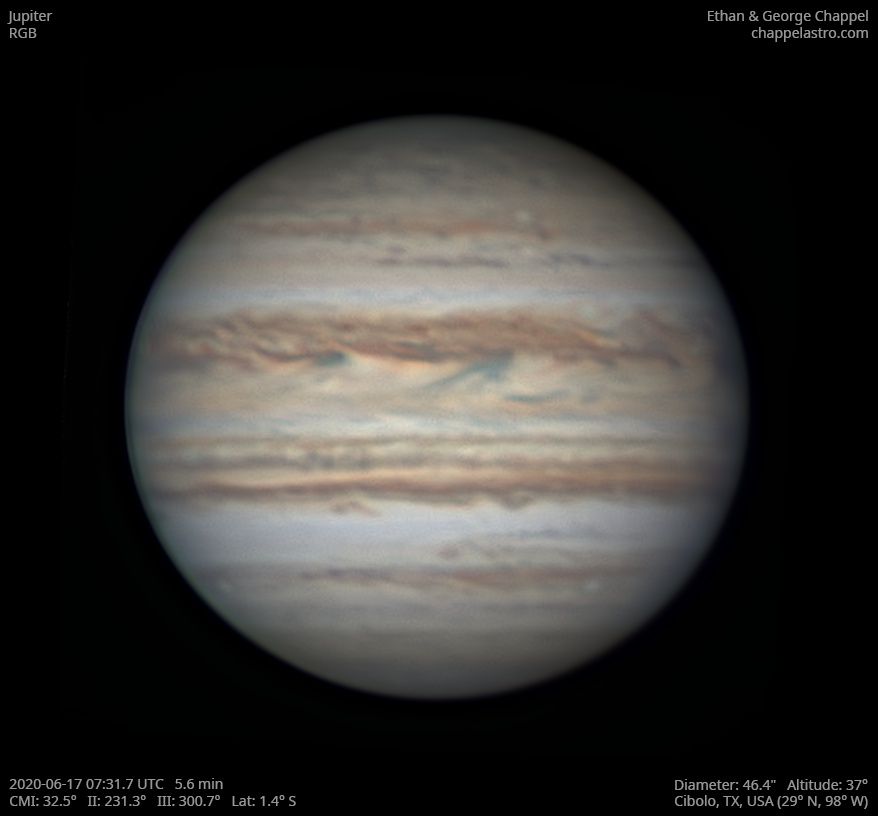# Jupiter 2020-06-17 07:31 UTC

CM1: 32.50°

CM2: 231.30°

CM3: 300.70°

CLat: -1.40°

Description

A second morning of fairly good seeing. It turns out the root of my motor focus problems was the serial cable connecting it to the controller. A new one was supposed to arrive a couple days ago, but the envelope was empty because it was improperly sealed!

Notes:

• The NEB is thinner on this hemisphere.
• White Spot Z has an orange perimeter.
• There are two small storms approaching the central meridian on the boundary between the SEB and STrZ.

Equipment

ZWO ASI290MM

Celestron EdgeHD 14

Logs
```FireCapture v2.6  Settings
------------------------------------
Observer=Ethan Chappel
Location=Cibolo
Scope=Celestron C14 EdgeHD
Camera=ZWO ASI290MM
Filter=R
Profile=Jupiter
Diameter=46.29"
Magnitude=-2.66
CMI=31.2° CMII=230.0° CMIII=299.4°  (during mid of capture)
FocalLength=7900mm (F/22)
Resolution=0.08"
Filename=2020-06-17-0729_9-EC-R-Jup.ser
Date=2020_06_17
Start=07_28_59.893
Mid=07_29_59.898
End=07_30_59.903
Start(UT)=07_28_59.893
Mid(UT)=07_29_59.898
End(UT)=07_30_59.903
Duration=120.010s
Date_format=yyyy_MM_dd
Time_format=HH_mm_ss
LT=UT -6h
Frames captured=7988
File type=SER
Binning=no
ROI=728x686
ROI(Offset)=128x56
FPS (avg.)=66
Shutter=15.00ms
Gain=300 (50%)
SoftwareGain=10 (off)
AutoHisto=75 (off)
Brightness=1 (off)
Gamma=50 (off)
USBTraffic=50 (off)
AutoGain=off
FPS=100 (off)
AutoExposure=off
HighSpeed=on
Histogramm(min)=0
Histogramm(max)=174
Histogramm=68%
Noise(avg.deviation)=2.79
Limit=120 Seconds
Sensor temperature=29.1°C
FireCapture v2.6  Settings
------------------------------------
Observer=Ethan Chappel
Location=Cibolo
Scope=Celestron C14 EdgeHD
Camera=ZWO ASI290MM
Filter=B
Profile=Jupiter
Diameter=46.29"
Magnitude=-2.66
CMI=33.4° CMII=232.2° CMIII=301.6°  (during mid of capture)
FocalLength=7950mm (F/22)
Resolution=0.08"
Filename=2020-06-17-0733_5-EC-B-Jup.ser
Date=2020_06_17
Start=07_32_34.778
Mid=07_33_34.780
End=07_34_34.783
Start(UT)=07_32_34.778
Mid(UT)=07_33_34.780
End(UT)=07_34_34.783
Duration=120.005s
Date_format=yyyy_MM_dd
Time_format=HH_mm_ss
LT=UT -6h
Frames captured=7897
File type=SER
Binning=no
ROI=728x686
ROI(Offset)=128x56
FPS (avg.)=65
Shutter=15.00ms
Gain=350 (58%)
SoftwareGain=10 (off)
AutoHisto=75 (off)
Brightness=1 (off)
Gamma=50 (off)
USBTraffic=50 (off)
AutoGain=off
FPS=100 (off)
AutoExposure=off
HighSpeed=on
Histogramm(min)=0
Histogramm(max)=71
Histogramm=27%
Noise(avg.deviation)=2.35
Limit=120 Seconds
Sensor temperature=30.0°C
FireCapture v2.6  Settings
------------------------------------
Observer=Ethan Chappel
Location=Cibolo
Scope=Celestron C14 EdgeHD
Camera=ZWO ASI290MM
Filter=G
Profile=Jupiter
Diameter=46.29"
Magnitude=-2.66
CMI=32.2° CMII=231.0° CMIII=300.5°  (during mid of capture)
FocalLength=7900mm (F/22)
Resolution=0.08"
Filename=2020-06-17-0731_6-EC-G-Jup.ser
Date=2020_06_17
Start=07_31_08.931
Mid=07_31_40.343
End=07_32_11.756
Start(UT)=07_31_08.931
Mid(UT)=07_31_40.343
End(UT)=07_32_11.756
Duration=62.825s
Date_format=yyyy_MM_dd
Time_format=HH_mm_ss
LT=UT -6h
Frames captured=6281
File type=SER
Binning=no
ROI=728x686
ROI(Offset)=128x56
FPS (avg.)=99
Shutter=10.00ms
Gain=335 (55%)
SoftwareGain=10 (off)
AutoHisto=75 (off)
Brightness=1 (off)
Gamma=50 (off)
USBTraffic=50 (off)
AutoGain=off
FPS=100 (off)
AutoExposure=off
HighSpeed=on
Histogramm(min)=0
Histogramm(max)=166
Histogramm=65%
Noise(avg.deviation)=3.23
Limit=120 Seconds
Sensor temperature=29.6°C
```